Home > CCA2 > Chapter Ch10 > Lesson 10.2.1 > Problem10-100

10-100.
1. Solve each of the following equations. Homework Help ✎

1.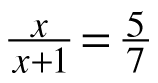2.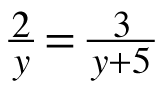3.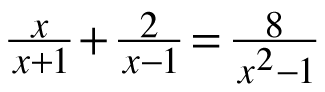4.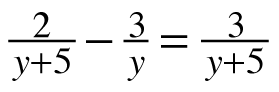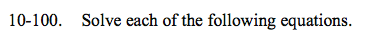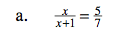See the help for problem 9-108.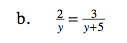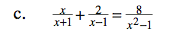x = −3, 2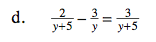$y=\frac{15}{4}$